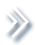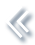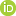####ArchivesReview Articles

## On finite mixture models

Jiahua Chen

Research Institute of Big Data, Yunnan University, Yunnan, China; Department of Statistics, University of British Columbia, Vancouver, Canadajhchen@stat.ubc.ca

Pages 15-27 | Received 15 Mar. 2017, Accepted 19 Apr. 2017, Published online: 12 May. 2017,
• Abstract
• References
• Citations

Finite mixture models are widely used in scientific investigations. Due to their non-regularity, there are many technical challenges concerning inference problems on various aspects of the finite mixture models. After decades of effort by statisticians, substantial progresses are recorded recently in characterising large sample properties of some classical inference methods when applied to finite mixture models, providing effective numerical solutions for mixture model-based data analysis, and the invention of novel inference approaches. This paper aims to provide a comprehensive summary on large sample properties of some classical statistical methods and recently developed modified likelihood ratio test and EM-test for the order of the finite mixture model. The presentation de-emphasises the rigour in order to gain some insights behind some complex technical issues. The paper wishes to recommend the EM-test as the most promising approach to data analysis problems from all models with mixture structures.

• Baum, L. E., Petrie, T., Soules, G., & Weiss, N. (1970). A maximization technique occurring in the statistical analysis of probabilistic functions of Markov chains. The Annals of Mathematical Statistics, 41(1), 164171[Google Scholar]
• Böhning, D. (2000). Computer-assisted analysis of mixtures and applications: Meta-analysis, disease mapping and others. London, UK: Chapman and Hall. [Google Scholar]
• Chen, H., & Chen, J. (2001). The likelihood ratio test for homogeneity in finite mixture models. Canadian Journal of Statistics, 29(2), 201215[Google Scholar]
• Chen, H., & Chen, J. (2003). Tests for homogeneity in normal mixtures in the presence of a structural parameter. Statistica Sinica, 13, 351365[Google Scholar]
• Chen, H., Chen, J., & Kalbfleisch, J. D. (2001). A modified likelihood ratio test for homogeneity in finite mixture models. Journal of the Royal Statistical Society: Series B, 63(1), 1929[Google Scholar]
• Chen, H., Chen, J., & Kalbfleisch, J. D. (2004). Testing for a finite mixture model with two components. Journal of the Royal Statistical Society: Series B, 66, 95115[Google Scholar]
• Chen, J. (1995). Optimal rate of convergence for finite mixture models. The Annals of Statistics, 23, 221233[Google Scholar]
• Chen, J. (1998). Penalized likelihood-ratio test for finite mixture models with multinomial observations. Canadian Journal of Statistics, 26(4), 583599[Google Scholar]
• Chen, J. (2016). Consistency of the mle under mixture models. Statistical Science. arXiv: 1607.01251. [Google Scholar]
• Chen, J., & Cheng, P. (2000). The limiting distribution of the restricted likelihood ratio statistic for finite mixture models. Chinese Journal of Applied Probability and Statistics, 2, 159–167[Google Scholar]
• Chen, J., Huang, Y., & Wang, P. (2016). Composite likelihood under hidden Markov model. Statistica Sinica, 26(4), 15691586[Google Scholar]
• Chen, J., & Li, P. (2011). Tuning the em-test for finite mixture models. Canadian Journal of Statistics, 39(3), 389404[Google Scholar]
• Chen, J., & Li, P. (2016). Testing the order of a normal mixture in mean. Communications in Mathematics and Statistics, 4(1), 2138[Google Scholar]
• Chen, J., Li, P., & Fu, Y. (2012). Inference on the order of a normal mixture. Journal of the American Statistical Association, 107(499), 10961105[Taylor & Francis Online]
• Chen, J., Tan, X., & Zhang, R. (2008). Inference for normal mixtures in mean and variance. Statistica Sinica, 18, 443465[Google Scholar]
• Chernoff, H., & Lander, E. (1995). Asymptotic distribution of the likelihood ratio test that a mixture of two binomials is a single binomial. Journal of Statistical Planning and Inference, 43(1), 1940[Google Scholar]
• Dacunha-Castelle, D., & Gassiat, E. (1999). Testing the order of a model using locally conic parametrization: Population mixtures and stationary arma processes. The Annals of Statistics, 27(4), 11781209[Google Scholar]
• Dannemann, J., & Holzmann, H. (2008a). Likelihood ratio testing for hidden Markov models under non-standard conditions. Scandinavian Journal of Statistics, 35(2), 309321[Google Scholar]
• Dannemann, J., & Holzmann, H. (2008b). Testing for two states in a hidden Markov model. Canadian Journal of Statistics, 36(4), 505520[Google Scholar]
• Davies, R. B. (1977). Hypothesis testing when a nuisance parameter is present only under the alternative. Biometrika, 64(1), 247254[Google Scholar]
• Davies, R. B. (1987). Hypothesis testing when a nuisance parameter is present only under the alternative. Biometrika, 74(1), 3343[Google Scholar]
• Dempster, A. P., Laird, N. M., & Rubin, D. B. (1977). Maximum likelihood from incomplete data via the em algorithm. Journal of the Royal Statistical Society. Series B, 39, 138[Crossref]
• Engel, C. (1994). Can the Markov switching model forecast exchange rates? Journal of International Economics, 36(1–2), 151165[Google Scholar]
• Friedlander, Y., & Leitersdorf, E. (1995). Segregation analysis of plasma lipoprotein (a) levels in pedigrees with molecularly defined familial hypercholesterolemia. Genetic Epidemiology, 12(2), 129143[Google Scholar]
• Frühwirth-Schnatter, S. (2006). Finite mixture and Markov switching models. New York, NY: Springer Science & Business Media[Google Scholar]
• Ghosh, J. K., & Sen, P. K. (1985). On the asymptotic performance of the log likelihood ratio statistic for the mixture model and related results. In L. LeCam & R. A. Olshen’ (Eds.), Proceedings of the Berkeley conference in honor of Jerzy Neyman and Jack Kiefer (Vol. 2, pp. 789806). Belmont, CA: Wadsworth[Google Scholar]
• Hamilton, J. D. (2010). Regime switching models. In Macroeconometrics and time series analysis (pp. 202209). London, UK: Palgrave Macmillan. [Google Scholar]
• Hartigan, J. A. (1985). A failure of likelihood asymptotics for normal mixtures. In L. LeCam & R. A. Olshen’ (Eds.), Proceedings of the Berkeley conference in honor of Jerzy Neyman and Jack Kiefer (Vol. 2, pp. 807810). Belmont, CA: Wadsworth[Google Scholar]
• Hathaway, R. J. (1985). A constrained formulation of maximum-likelihood estimation for normal mixture distributions. The Annals of Statistics, 13, 795800[Google Scholar]
• Holzmann, H., & Schwaiger, F. (2016). Testing for the number of states in hidden Markov models. Computational Statistics and Data Analysis, 100, 318330[Google Scholar]
• Kiefer, J., & Wolfowitz, J. (1956). Consistency of the maximum likelihood estimator in the presence of infinitely many incidental parameters. The Annals of Mathematical Statistics, 27, 887906[Google Scholar]
• Lemdani, M., & Pons, O. (1999). Likelihood ratio tests in contamination models. Bernoulli, 5(4), 705719[Google Scholar]
• Li, P., & Chen, J. (2010). Testing the order of a finite mixture. Journal of the American Statistical Association, 105(491), 10841092[Taylor & Francis Online]
• Li, P., Chen, J., & Marriott, P. (2009). Non-finite fisher information and homogeneity: An EM approach. Biometrika, 96, 411426[Google Scholar]
• Li, S., Chen, J., Guo, J., Jing, B.-Y., Tsang, S.-Y., & Xue, H. (2015). Likelihood ratio test for multi-sample mixture model and its application to genetic imprinting. Journal of the American Statistical Association, 110(510), 867877[Taylor & Francis Online]
• Lindsay, B. G. (1988). Composite likelihood methods. Contemporary Mathematics, 80(1), 221239[Google Scholar]
• Lindsay, B. G. (1995). Mixture models: Theory, geometry and applications. In NSF-CBMS regional conference series in probability and statistics. Hayward, CA: Institute of Mathematical Statistics[Google Scholar]
• Liu, X., & Shao, Y. (2003). Asymptotics for likelihood ratio tests under loss of identifiability. Annals of Statistics, 31, 807832[Google Scholar]
• McLachlan, G., & Peel, D. (2004). Finite mixture models. New York, NY: John Wiley & Sons[Google Scholar]
• Morris, C. N. (1982). Natural exponential families with quadratic variance functions. The Annals of Statistics, 10, 6580[Google Scholar]
• Neyman, J., & Scott, E. (1965). On the use of c (alpha) optimal tests of composite hypotheses. Bulletin of the International Statistical Institute, 41(1), 477497[Google Scholar]
• Niu, X., Li, P., & Zhang, P. (2011). Testing homogeneity in a multivariate mixture model. Canadian Journal of Statistics, 39(2), 218238[Google Scholar]
• Ott, J. (1999). Analysis of human genetic linkage. Baltimore, MD: JHU Press[Google Scholar]
• Pearson, K. (1894). Contributions to the mathematical theory of evolution. Philosophical Transactions of the Royal Society of London A, 185, 71110[Google Scholar]
• Schork, N. J., Allison, D. B., & Thiel, B. (1996). Mixture distributions in human genetics research. Statistical Methods in Medical Research, 5(2), 155178[Google Scholar]
• Shen, J., & He, X. (2015). Inference for subgroup analysis with a structured logistic-normal mixture model. Journal of the American Statistical Association, 110(509), 303312[Taylor & Francis Online]
• Titterington, D. M., Smith, A. F., & Makov, U. E. (1985). Statistical analysis of finite mixture distributions. New York, NY: Wiley[Google Scholar]
• Varin, C. (2008). On composite marginal likelihoods. AStA – Advances in Statistical Analysis, 92(1), 128[Google Scholar]
• Varin, C., Reid, N., & Firth, D. (2011). An overview of composite likelihood methods. Statistica Sinica, 21, 542[Google Scholar]
• Wilks, S. S. (1938). The large-sample distribution of the likelihood ratio for testing composite hypotheses. The Annals of Mathematical Statistics, 9(1), 6062[Google Scholar]
• Wu, C. J. (1983). On the convergence properties of the em algorithm. The Annals of statistics, 11, 95103[Google Scholar]

Walaa A. El-Sharkawy, Moshira A. Ismail. (2020) Mixture of Birnbaum-Saunders Distributions: Identifiability, Estimation and Testing Homogeneity with Randomly Censored Data. American Journal of Mathematical and Management Sciences 0:0, pages 1-16.

Kuo-Jung Lee, Ray-Bing Chen. (2019) Bayesian variable selection in a finite mixture of linear mixed-effects models. Journal of Statistical Computation and Simulation 89:13, pages 2434-2453.